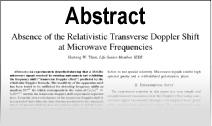Enter the content which will be displayed in sticky bar

AbstractThe Electromagnetic Momentum of a Charged Particle in Weber?s Theory

Year: 1997
In the Lagrangian formulation of the Lorentz force between two moving charged particles, particle momentum is shown to include a term proportional to the vector potential in addition to the usual mechanical momentum of mass times velocity. This additional term is sometimes referred to as the electromagnetic momentum of the particle. (It is not the same as the momentum of the electromagnetic field.) In this paper the Weber force is put into Lagrangian form and an electromagnetic momentum term appears. The electromagnetic momentum occurring in Weber\'s theory is compared with that of the Lorentz force. Neither electromagnetic momentum has an intuitive physical explanation and both might be only mathematical artifacts arising from Lagrangian dynamics.